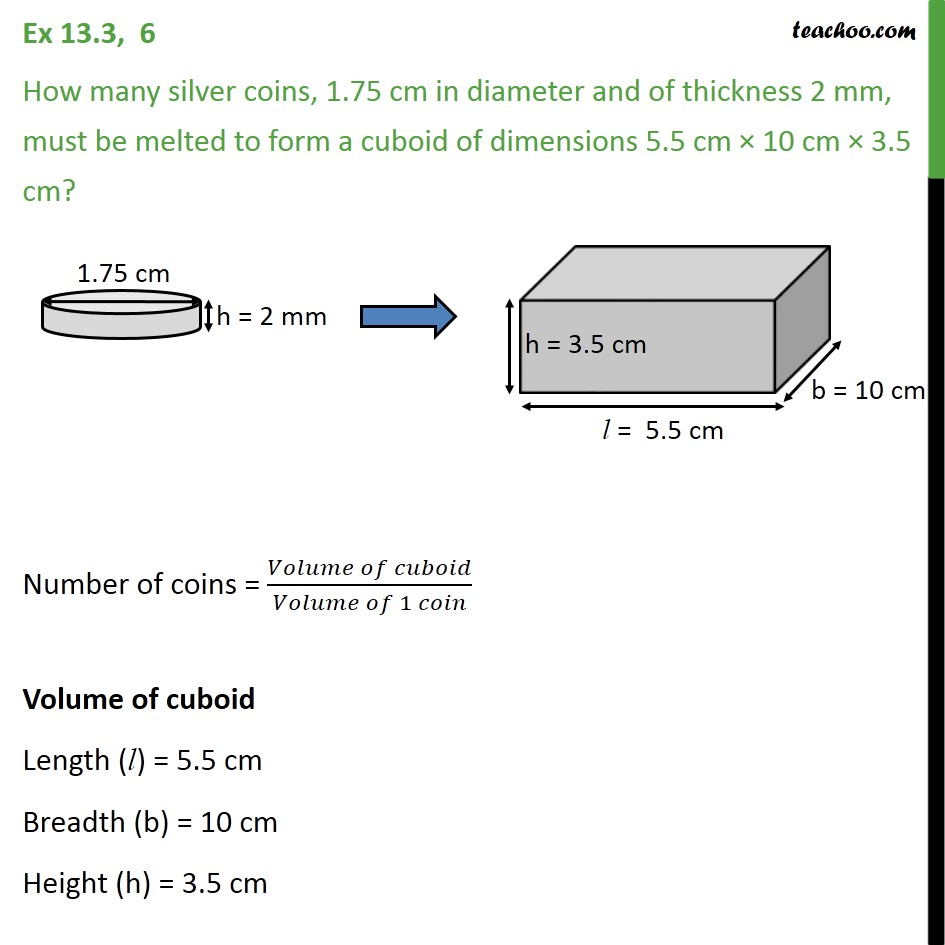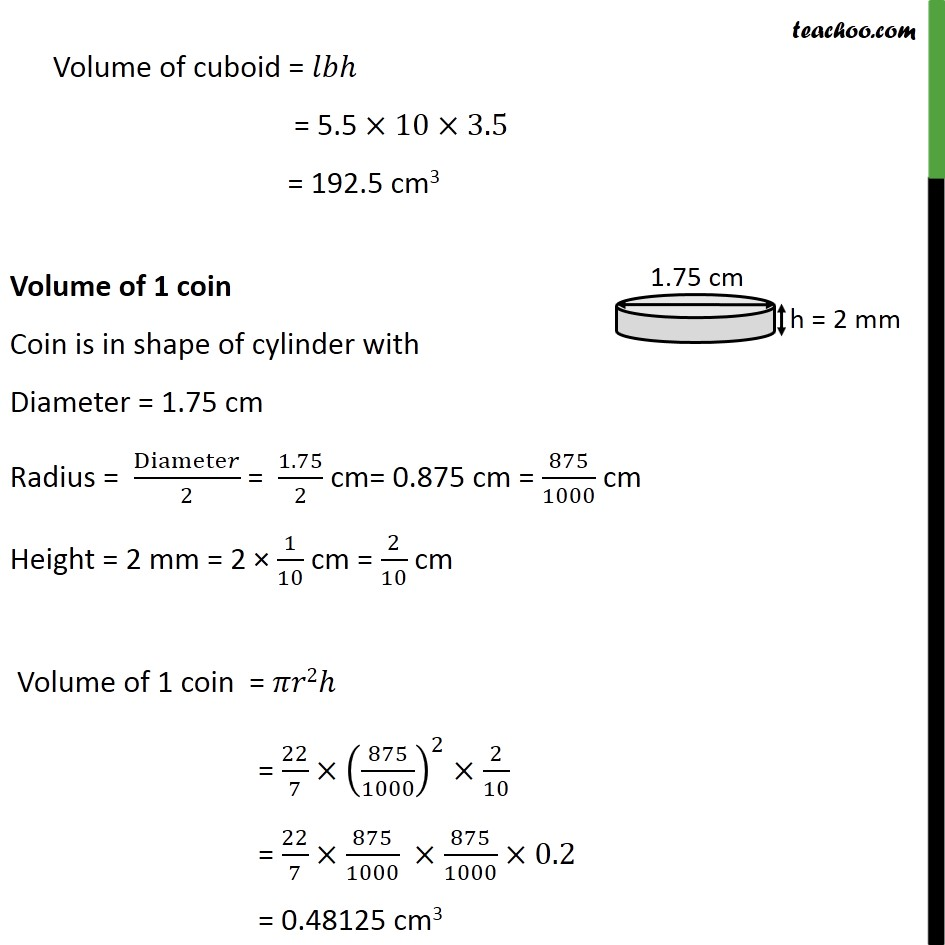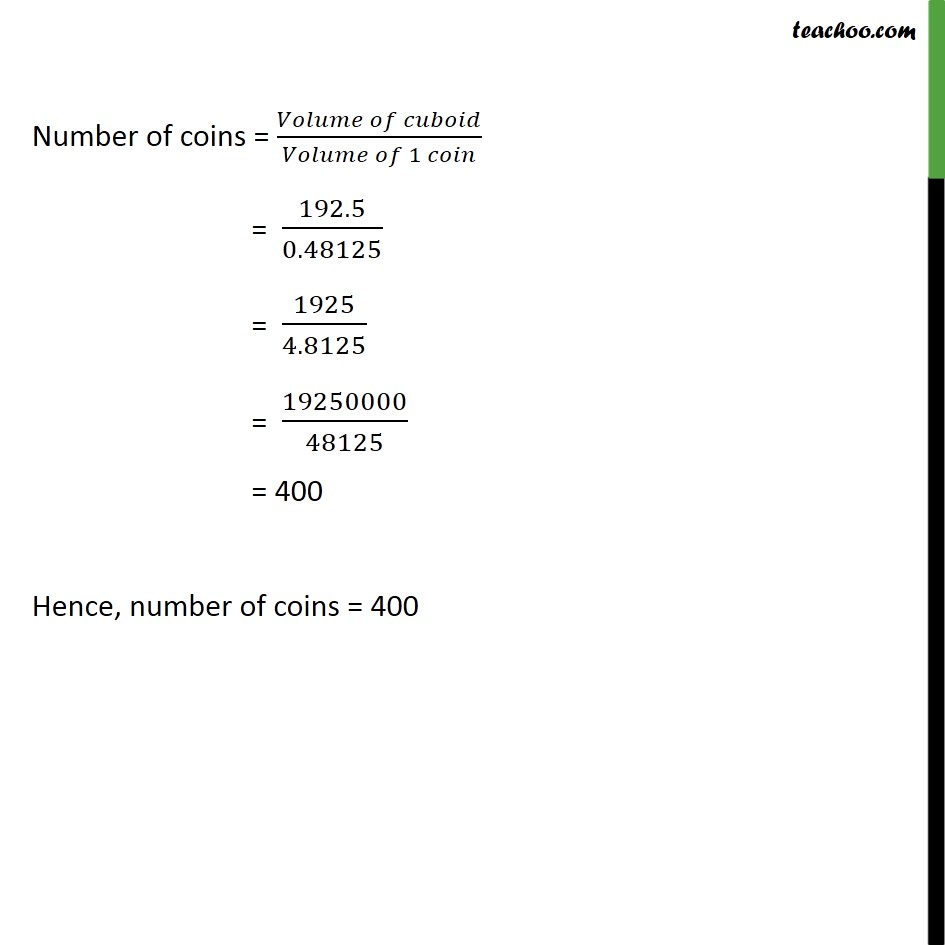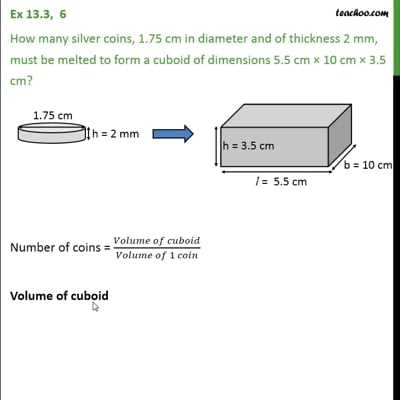Ex 13.3

Chapter 13 Class 10 Surface Areas and Volumes
Serial order wiseThis video is only available for Teachoo black users

Maths Crash Course - Live lectures + all videos + Real time Doubt solving!

### Transcript

Ex 13.3, 6 How many silver coins, 1.75 cm in diameter and of thickness 2 mm, must be melted to form a cuboid of dimensions 5.5 cm 10 cm 3.5 cm? Number of coins = ( )/( 1 ) Volume of cuboid Length (l) = 5.5 cm Breadth (b) = 10 cm Height (h) = 3.5 cm Volume of cuboid = = 5.5 10 3.5 = 192.5 cm3 Volume of 1 coin Coin is in shape of cylinder with Diameter = 1.75 cm Radius = Diamete /2 = 1.75/2 cm= 0.875 cm = 875/1000 cm Height = 2 mm = 2 1/10 cm = 2/10 cm Volume of 1 coin = 2 = 22/7 (875/1000)^2 2/10 = 22/7 875/1000 875/1000 0.2 = 0.48125 cm3 Volume of cuboid = 𝑙𝑏ℎ = 5.5 ×10×3.5 = 192.5 cm3 Volume of 1 coin Coin is in shape of cylinder with Diameter = 1.75 cm Radius = Diamete𝑟/2 = 1.75/2 cm= 0.875 cm = 875/1000 cm Height = 2 mm = 2 × 1/10 cm = 2/10 cm Volume of 1 coin = 𝜋𝑟2ℎ = 22/7×(875/1000)^2× 2/10 = 22/7×875/1000 ×875/1000×0.2 = 0.48125 cm3 Volume of cuboid = 𝑙𝑏ℎ = 5.5 ×10×3.5 = 192.5 cm3 Volume of 1 coin Coin is in shape of cylinder with Diameter = 1.75 cm Radius = Diamete𝑟/2 = 1.75/2 cm= 0.875 cm = 875/1000 cm Height = 2 mm = 2 × 1/10 cm = 2/10 cm Volume of 1 coin = 𝜋𝑟2ℎ = 22/7×(875/1000)^2× 2/10 = 22/7×875/1000 ×875/1000×0.2 = 0.48125 cm3 Number of coins = ( )/( 1 ) = 192.5/0.48125 = 1925/4.8125 = 19250000/48125 = 400 Hence, number of coins = 400